###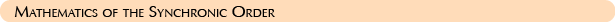At its source, the synchronic order is pure mathematics; the mathematics of the fourth dimension. Number precedes consciousness. Number is supramental—beyond mind. Number is what gives mind its sense of order, harmony and organization. The synchronic order is based on a set of fourth-dimensional mathematical constants. The Galactic Compass demonstrates the complete mathematical principles of fourth-dimensional time.

The vastness of the universal order of time is knowable through simple mathematical codes and laws, the most basic being the ratio formulation: 4:7::7:13.  Coded within this formulation is the ratio of the universal frequency of synchronization: 13:20.

All numbers are composites and/or factors of each other. The primal numbers of time are 4, 7, 13 and 20. The primal numbers of space are 4, 5 and 9. The frequency of the Law of Time is 13:20—between the 13 and 20 is the seven. Between 3 and 7 is the four. Herein lies the form constant that establishes the ratio 4:7::7:13. Four is the root, seven is the stem and thirteen is the fruit. This is the primal ratio and cosmology of the Law of Time.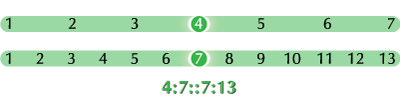In this construct, there are three units on either side of the seven—this is the underlying law of three. 3 + 3 = 6. Six is the basis of the hexagisimal order.

3 + 1 + 3 = 7;
6 + 1 + 6 = 13.

Parallel constructs can be derived from doubling the law of three. So we have the 7, 13 and 20. The point is that the fundamental formulation of the numbers and patterns in time is contained in this formulation.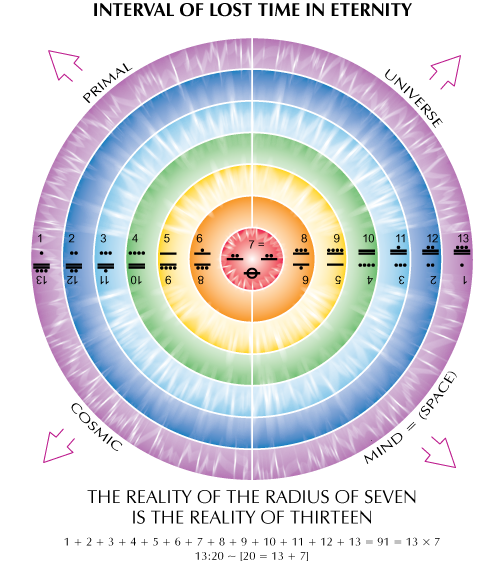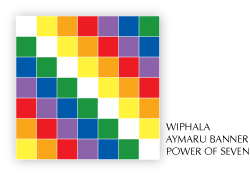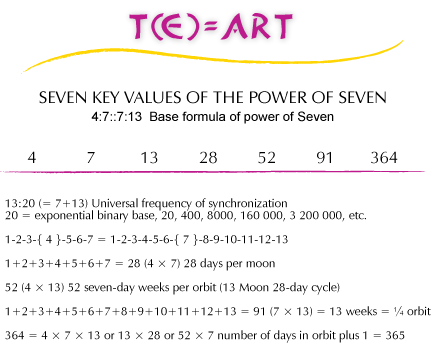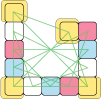Particularly with the seven and the thirteen we are dealing with supremely cosmic frequencies, the 7 being derived from the interval of lost time in eternity and the 13 being the function of the 4:7::7:13 that creates endless cosmic cyclical patterns. The 13 then forms into a type of spiral which is known as the wavespell. By comparison, space is a static structure.

1 + 2 + 3 + 4 = 10. Ten is the decimal base.
7 + 13 = 20. Twenty is the vigesimal base.

Fourth-dimensional time is based on 20 and third-dimensional space (as matter) is based on 10. That ratio difference is what creates the higher etheric patterns of time in relation to the denser patterns of space.

Dynamic Numbers of Time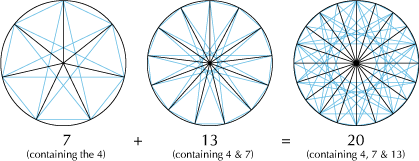Static Numbers of SpaceSymbolic structures are derived from the primary numbers of time: 4, 7, 13, and 20. The number 20 (4 x 5) actually incorporates a fifth force of space. In other words, the 20 is a function of time (7 and 13). It is also a function of space (4 x 5). When we multiply the space numbers 4 x 5, we arrive at the 20—a prime number of time. Therefore 20 is the magic number that comprises both space and time.  This is why twenty is known as the number of totality and 20 squared is 400, the pure fractal frequency of totality.

In the vigesimal system, 400 is written as 1.0.0, there are zero units in the 1-unit and 20- unit positions, and a 1 in the 400-unit position. This is the totality. The square of the totality of time (400) is four times greater than the square of the totality of space (100). Yet 1.0.0 vigesimal looks like 100 decimal.

error: Content is protected !!